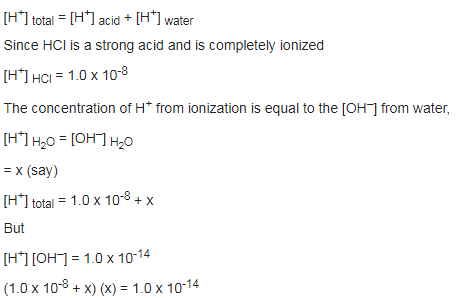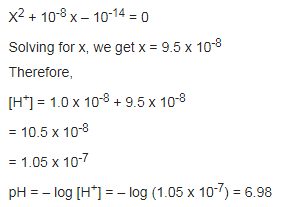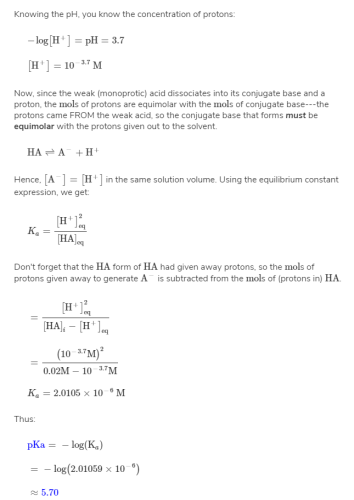Courses

# Test: Ionisation Of Water

## 10 Questions MCQ Test Chemistry Class 11 | Test: Ionisation Of Water

Description
This mock test of Test: Ionisation Of Water for Class 11 helps you for every Class 11 entrance exam. This contains 10 Multiple Choice Questions for Class 11 Test: Ionisation Of Water (mcq) to study with solutions a complete question bank. The solved questions answers in this Test: Ionisation Of Water quiz give you a good mix of easy questions and tough questions. Class 11 students definitely take this Test: Ionisation Of Water exercise for a better result in the exam. You can find other Test: Ionisation Of Water extra questions, long questions & short questions for Class 11 on EduRev as well by searching above.
QUESTION: 1

### Which of the following is more acidic? A solution with pH 5 or a solution with pH 3

Solution:

We know that pH equals to - log[H+] or the value of pH is inversely proportional to the concentration of H+.  So more the value of pH, less the solution be acidic.

QUESTION: 2

Solution:
QUESTION: 3

### Which one of the following is the approximate pH of 0.01 M solution of NaOH at 298 k?

Solution:

First off, since NaOH is a strong base, it will dissociate completely into Na+ and OH-. Thus, we know that we have 0.01 M OH-.

However, we do not know anything about the concentration of H+. Fortunately, we do not need to, as pH + pOH = 14. So, if we find pOH, we can solve for pH. p is a mathematical function equivalent to -log. So, pH actually means -log[H+] (Note that brackets indicate concentration of).

pOH = -log 0.01M OH-

pOH = 2

pH + 2 = 14

pH = 12

This result makes sense, since a solution of strong base should have a high pH.

QUESTION: 4

A 0.10 M solution of a weak acid, HX, is 0.059% ionized. Evaluate Ka for the acid.

Solution:

Since the acid is only 0.059% ionized, therefore the concentration of ions in solution = 0.1 x 0.059 / 100 = 0.000059

Ka = [H+] [X] / [HX] = (0.000059)2 / 0.1 = 3.5 x 10-8

QUESTION: 5

In a sample of pure water, only one of the following statements is always true at all conditions of temperature and pressure.

Solution:
QUESTION: 6

The pH of 10-8 molar solution of HCl in water is:

Solution:

If we use the relation, pH = – log [H3O+], we get pH equal to 8. But this is not correct because an acidic solution cannot have pH greater than 7. It may be noted that in very dilute acidic solution, when H+ concentrations from acid and water are comparable, the concentration of H+ from water cannot be neglected.

Therefore,QUESTION: 7

Which one of the following is correct for a solution in which [H+] = 10-8 M.

Solution:
QUESTION: 8

Which one of the following is a wrong derivation?

Solution:
QUESTION: 9

Value of pH is determined by

Solution: The pH measurement electrode is made of special glass which, due to its surface properties, is particularly sensitive to hydrogen ions. The pH measurement electrode is filled with a buffer solution which has a pH value of 7.
QUESTION: 10

The pH of a 0.02 M solution of an unknown weak acid is 3.7. What is the pKa of this acid?

Solution: